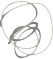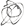Noppanit

16 Jan 2016

# Round to the left most digit

It’s probably unusual for me to write anything about Math. I hated math and I failed the subject all the time. But I got pretty excited when I knew this trick from my colleague. I also asked this question at stackoverflow.

### The problem

Let’s say you have a number 423 and you want to round the number to the nearest left-most digit which in this case it’s 4. If you want to round to the left-most digit it’s going to be 400.

### The solution

The solution is quiet simple. You just need to get the place value of the number, take the number in question divided by the place value and floor the number then multiply the result with the place value again. That’s it! Simple right?

So, the place value of 423 is 100.

\begin{align} \frac{423}{100} \end{align}

which you will get 4.23. The you floor the number

  Math.floor(4.23); // 4


And then you multiple 4 with 100 to get the rounded number.

\begin{align} {400}\cdot{100} = {400} \end{align}

The problem is how are we going to get the place value of the number?

You want logarithm.

The idea of this is to reverse the operation of exponentiation. For example, the log10 of 423 is 2.62634036738 then 10^2.62634036738 equals 423. But you want the place value. You would need to round the 2.62634036738 which is going to be 2 then 10^2 is 100. There! you get the place value of the 4.

\begin{align} d = \lfloor\frac{n}{10^{\lfloor\log_{10} n \rfloor}} \rfloor \cdot 10^{\lfloor\log_{10} n\rfloor} \end{align}

### Show me the code

n = 423
d = Math.floor(Math.log10(n))

Math.floor(n / Math.pow(10, d)) * Math.pow(10, d) // 400


Til next time,
noppanit at 17:30